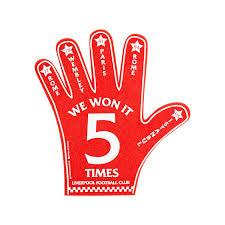# Increased 2722

Five times the number is the same as three times increased by 14. What is the number?

x =  7

### Step-by-step explanation:

5x = 3x + 14

5·x = 3·x + 14

2x = 14

x = 14/2 = 7

x = 7

Our simple equation calculator calculates it.Did you find an error or inaccuracy? Feel free to write us. Thank you!

Tips for related online calculators
Do you have a linear equation or system of equations and looking for its solution? Or do you have a quadratic equation?